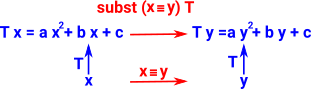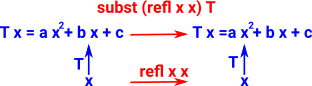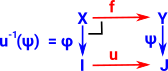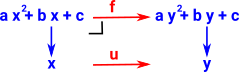# Equality Rules

There are rules for both definitionally equality and propositional equality

### Rules for Definitionally Equality

The rules must be normalising so,

• All rules must reduce the expression in some way as the rules are applied automatically and there must be no possibility of a loop where rules get applied indefinitely.
• The evaluation of an expression containing only literal values will give a value.
• There may also be rules for expressions containing variables as long as these rules are sure to terminate but these rules may be more ad hoc. For example, in Idris function parameters that are not case-split may be reduced.

As an example, a rule for symmetry x=y -> y=x can't be a definitionally equality rule and so must be a propositional equality rule because:

• It may not be necessary.
• If applied automatically it would be called indefinately.

### Rules for Propositional Equality

The rules are used to prove equality so its harder to have some automatic algorithm and so the programmer needs to apply the rules as required to do the proof.

### Substitution

There seems to be at least 2 sorts of rules we can apply to equations:

• Change variable to another variable or expression (provided we do it consistently everywhere it occurs, including in types and in context)
• Replace like for like.

If we substitute, in a term M all occurrences of
context variable x by a term N of the same type
as x, then the result is the same type - it will remain true.

 ΓN:σ Γ,x:σ, ΔM Γ,Δ[N/x]M[N/x]
 example where: N= y+1 M = a x² + b x + cSubstitution happens in function application (that is, when deconstructing a function. See β-reduction in λ-calulus. (λ x:σ.N) M

#### Definition of 'subst'.

subst takes T x to T y.

We can think of T x as being an expression in x. So subst takes an expression in x to an expression in y.q:(x=y)T : A -> Typet :T x subst q T t : T yBelow is the computational rule where the two values are already definitionaly equal. Here refl (propositional equality) is embedded into this definitionaly equality.

 (subst (refl x x) T t)t : T xAlso we can replace like for like in equations (is this substitution?).

#### Rewriting in Logic

Rewriting an expression into a logically equivalent one.

example: ¬A /\ ¬B -> ¬(A \/ B)

#### Computation rule

Refl disappears when properties are definitionaly equal.

 subst (Refl P)P

 Substitution in Agda ```id:{A: Set}->A->A _==_:{A: Set}->A->A->Set subst:{A: Set} (C:A->Set) {x y:A}->x==y->C x->C y``` ```subst : forall {x y} -> x == y -> x -> y subst refl x = x```

 Substitution in Idris (replace) ```||| Perform substitution in a term according to some equality. replace : {a:_} -> {x:_} -> {y:_} -> {P : a -> Type} -> x = y -> P x -> P y replace Refl prf = prf```

#### Substitution as a fibrationexampleWhere I can, I have put links to Amazon for books that are relevant to the subject, click on the appropriate country flag to get more details of the book or to buy it from them.Computation and Reasoning - This book is about type theory. Although it is very technical it is aimed at computer scientists, so it has more discussion than a book aimed at pure mathematicians. It is especially useful for the coverage of dependant types.